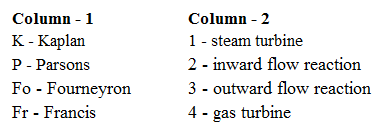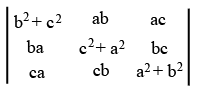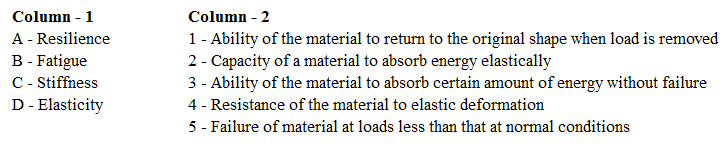# ISRO Scientist or Engineer Mechanical 2016

Instructions

For the following questions answer them individually

Question 71

# A wire has a mass ($$0.3 \pm 0.003$$) g, radius ($$0.5 \pm 0.005$$) mm and length ($$6 \pm 0.06$$) cm. The maximum percentage error in the measurementof density isQuestion 72

# Match the items in column-1 and column-2 and choose the correct combinationQuestion 73

# Which ofthe following pressure gauge is generally used for the calibration of other pressure measuring gaugesQuestion 74

# In a ball bearing, if the number of balls is reduced to half and diameter of the ball is increased to 4 times, then static load capacity of the ball bearing isQuestion 75

# The velocity of sound in winter compared to summer will beQuestion 76

# Gaseous Nitrogen at $$30^\circ C$$ is expanded through a converging nozzle from a total pressure of 0.5 MPa to a back pressure chamber.If the back pressure is increased from 0.1 MPa to 0.4 MPa in steps of 0.02 MPa, the trend in massflow rate through the nozzleQuestion 77

# What is the value ofQuestion 78

# Critical to Stagnation Pressure ratio $$\left(\frac{P^*}{P_0}\right)$$ for an expansion process isQuestion 79

# Match the items in column-1 and column-2 and choose the correct combinationQuestion 80

# A Pelton wheel turbine operates under a head of 125 m at a speed of 2000 rpm. To operate very close to the maximumefficiency, the mean diameter of the Pelton wheelis nearlyOR